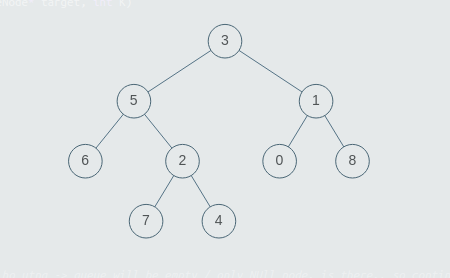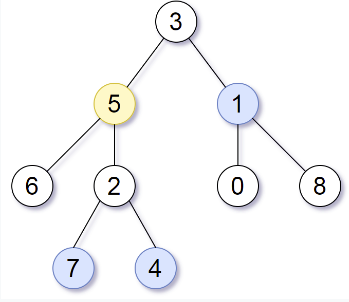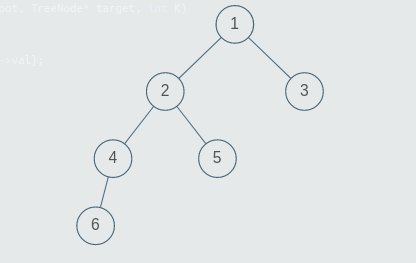3

Print Nodes at Distance K From a Given Node

Difficulty: HARD
Avg. time to solve
20 min
Success Rate
80%

Problem Statement

Note:

1. A binary tree is a tree in which each node has at most two children.
2. The given tree will be non-empty.
3. The given tree can have multiple nodes with the same value.
4. If there are no nodes in the tree which are at distance = K from the given node, return an empty list.
5. You can return the list of values of valid nodes in any order. For example if the valid nodes have values 1,2,3, then you can return {1,2,3} or {3,1,2} etc.
Input Format:
The first line of the input contains an integer T, denoting the number of test cases to run.

The first line of each test case will contain the values of the nodes of the tree in the level order form ( -1 for NULL node). Refer to the example below for further explanation.

The second line of each test case contains the value of the target node.

The third and the last line of each test case contains the integer K denoting the distance at which nodes are to be found.

Example:

Consider the binary tree:The input for the tree depicted in the above image would be :

3
5 1
6 2 0 8
-1 -1 7 4 -1 -1 -1 -1
-1 -1 -1 -1

Explanation :
Level 1 :
The root node of the tree is 3

Level 2 :
Left child of 3 = 5
Right child of 3 = 1

Level 3 :
Left child of 5 = 6
Right child of 5 = 2
Left child of 1 = 0
Right child of 1 = 8

Level 4 :
Left child of 6 = null (-1)
Right child of 6 = null(-1)
Left child of 2 = 7
Right child of 2 = 4
Left child of 0 = null (-1)
Right child of 0 = null (-1)
Left child of 8 = null (-1)
Right child of 8 = null (-1)

Level 5 :
Left child of 7 = null (-1)
Right child of 7 = null (-1)
Left child of 4 = null (-1)
Right child of 4 = null (-1)

The first not-null node (of the previous level) is treated as the parent of the first two nodes of the current level. The second not-null node (of the previous level) is treated as the parent node for the next two nodes of the current level and so on.

The input ends when all nodes at the last level are null (-1).
Output Format :
For each test case, print the values of all nodes at distance = K, from the given target node.

Note:

You do not need to print anything, it has already been taken care of. Just implement the given function.
Constraints:
1 <= T <= 100
1 <= N <= 3000
0 <= K <= 3000
0 <= nodeValue <= 3000

Where nodeValue donates the value of the node.

Time Limit: 1 sec
Sample Input 1 :
1
3 5 1 6 2 0 8 -1 -1 7 4 -1 -1 -1 -1 -1 -1 -1 -1
5
2
7 4 1
Explanation For The Sample Output 1:Target Node is 5. Nodes at distance 1 from 5 are {6, 2, 3} and nodes at distance 2 are {7, 4, 1}.
Sample Input 2:
1
1 2 3 4 5 -1 -1 6 -1 -1 -1 -1 -1
5
3
6 3
Explanation For The Sample Output 2:The tree will be generated as above. The target node is 5 and K = 3. The nodes at distance 1 from node 5 are {2}, nodes at distance 2 from node 5 are {1, 4} and nodes at distance 3 from node 5 are {6, 3}.Console